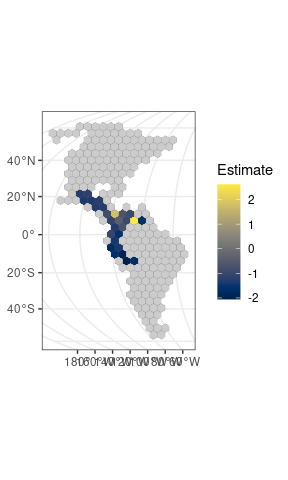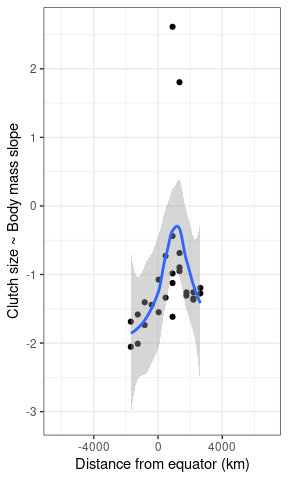# rangeMapper

### Packages and data

require(rangeMapper)
require(sf)
require(data.table)
require(ggplot2)
require(viridis)

data(dem)
wrens\$breeding_range_area = st_area(wrens)

### A bare bone rangeMapper project

The project contains nothing but several system tables.

# path is not specified so an in-memory file is created.
con = rmap_connect()

Wrens breeding ranges are imported.

rmap_add_ranges(con, x = wrens, ID = 'sci_name')
rmap_prepare(con, 'hex', cellsize = 500)
rmap_add_bio(con, wrens, 'sci_name')

### Do larger wren species have smaller clutches ?

lm(clutch_size~log(body_mass), wrens)  %>%  summary
#>
#> Call:
#> lm(formula = clutch_size ~ log(body_mass), data = wrens)
#>
#> Residuals:
#>      Min       1Q   Median       3Q      Max
#> -2.41154 -1.00417 -0.02835  0.99885  2.94120
#>
#> Coefficients:
#>                Estimate Std. Error t value Pr(>|t|)
#> (Intercept)      5.8573     1.2496   4.687 2.49e-05 ***
#> log(body_mass)  -0.8291     0.4183  -1.982   0.0534 .
#> ---
#> Signif. codes:  0 '***' 0.001 '**' 0.01 '*' 0.05 '.' 0.1 ' ' 1
#>
#> Residual standard error: 1.303 on 46 degrees of freedom
#>   (36 observations deleted due to missingness)
#> Multiple R-squared:  0.0787, Adjusted R-squared:  0.05868
#> F-statistic:  3.93 on 1 and 46 DF,  p-value: 0.05343

### Does the slope clutch size ~ body mass vary spatially?

First we save a species richness map.

rmap_save_map(con)

Then we construct a subset table with all assemblages with a richness of at least 10 species.

rmap_save_subset(con,'sset1', species_richness = 'species_richness > 10')

Now we can construct a clutch size ~ body mass map with assemblages containing at least 10 species.

linmod = function(x) {
lm(clutch_size ~ log(body_mass), x) %>%
summary %>% coefficients %>% data.table %>% .[-1] }

rmap_save_map(con, fun= linmod, subset= 'sset1', src='wrens', dst='slope_clutch_size')

We get the map as a sf data.frame and plot it with ggplot.

x = rmap_to_sf(con)

ggplot() +
geom_sf(data = x, aes(fill = Estimate),  size= 0.05) +
scale_fill_gradientn(colours =  viridis(10, option = 'E'), na.value= 'grey80') +
theme_bw()Here is the “answer” to the question above.

xy = st_centroid(x)  %>% st_coordinates
x = cbind(x, xy )

ggplot(x , aes(y = Estimate, x = Y) ) +
geom_point() +
geom_smooth() +
theme_bw() +
ylab('Clutch size ~ Body mass slope') +
xlab('Distance from equator (km)')